# Calculus 1 : Relative Minimum

## Example Questions

### Example Question #1 : Relative Minimum

The following is a list of values at certain points for continuous. By mean-value theorem, how many zeroes must this function have?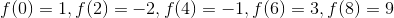Exactly 1

At least 2

At least 1

Exactly 2

At most 2

At least 2

Explanation:

The mean value theorem states that if continuoushas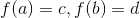, then for all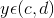, there must be anthat maps to, so since the value above go from negative to positive twice, zero must be mapped to at least twice.

### Example Question #2 : Relative Minimum

A relative minimum of a function is all the points x, in the domain of the function, such that it is the smallest value for some neighborhood.  These are points in which the first derivative is 0 or it does not exist.

Find the relative minimum of the function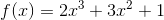using the following graph and the function.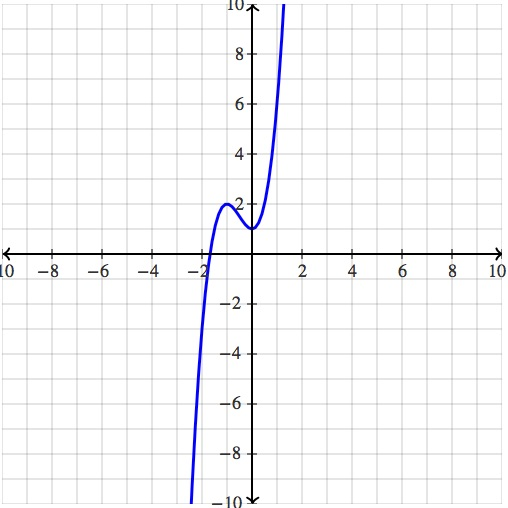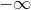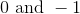Explanation:

We notice that this function has 2 extrema which are located at x=-1 and x=0.  We could have also of found this by looking at the function itself:.

We know that extrema exist when the slope of the function is zero, hence we take the derivative, set it equal to zero, and than solve for x.

The derivative is the following: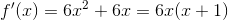.

Therefore setting both pieces equal to zero we see the following: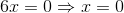and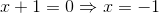.

Finding out if one of these x values produce a local min or max however requires either the first derivative test, the second derivative test, or analyzing the graph. We see that for a small neighborhood around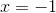,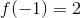is the largest term, hence it is a local max. Similarly, for a small neighborhood around,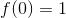is the smallest term, hence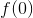is a local min and the only local min of this function. Remember that it is not the x value that must be the smallest, rather it is it's corresponding y value.

### All Calculus 1 Resources